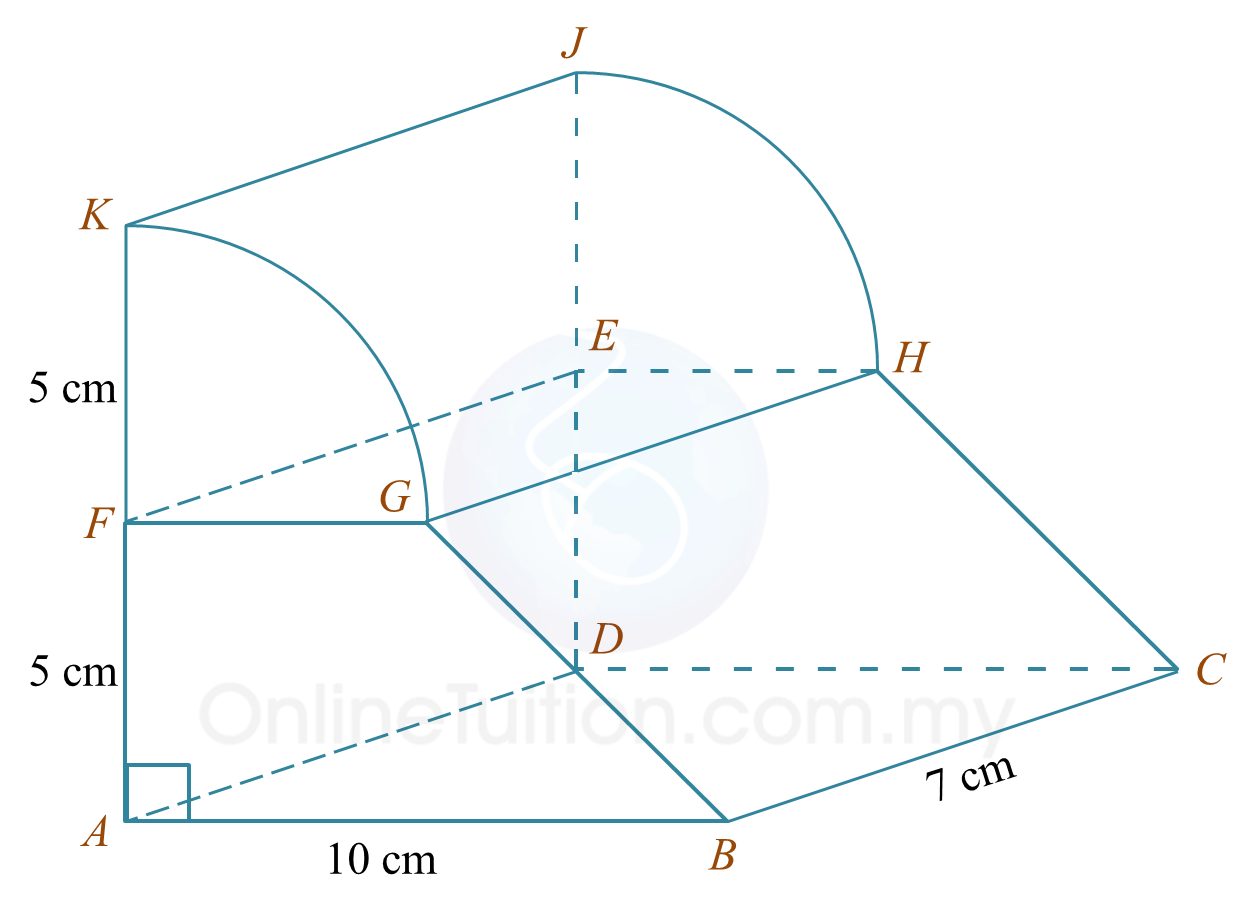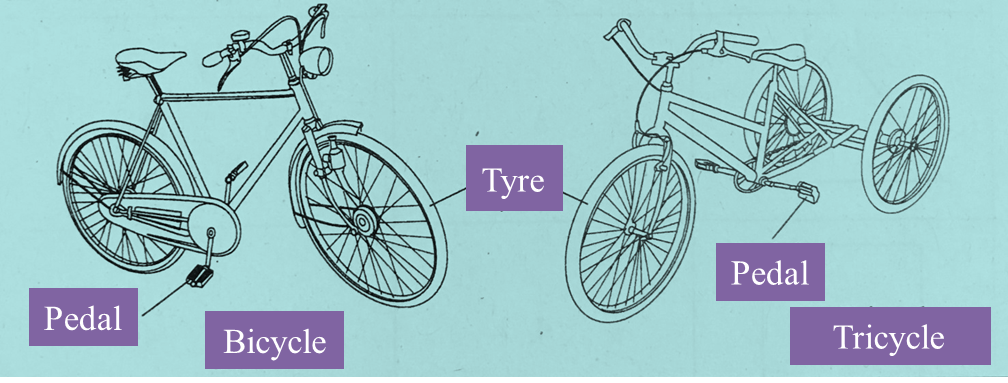# SPM Mathematics 2017, Paper 2 (Questions 3 & 4)

Question 3 (4 marks):
Diagram 2 shows a composite formed by joining a quarter cylinder and a right prism at the rectangular plane EFGH. The trapezium ABGF and the quarter circle FGK are the uniform cross sections of the solid.Diagram 2

Using  $\pi =\frac{22}{7}$ , calculate the volume, in  cm3, of the composite solid.

Solution:

Question 4 (5 marks):
Solution by matrix method is not allowed to answer this question.
Diagram 3 shows a bicycle and a tricycle.Diagram 3

Calculate the number of bicycles and the number of tricycles if there are 64 pedals and 74 tyres.

Solution:
Let bicycle = x and tricycle = y
So,
2x + 2y = 64 …………. (1)
2x + 3y = 74 …………. (2)
(2) – (1)
3y – 2y = 74 – 64
y = 10

When y = 10
From (2)
2x + 3(10) = 74
2x = 7430
2x = 44
x = 22

Thus, the number of bicycles are 22 and the number of tricycles are 10.